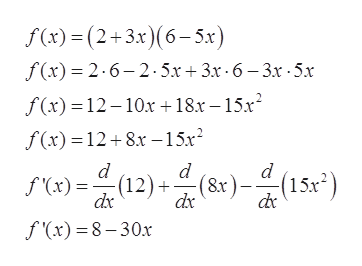# Find f'(x) and find the equation of the line tangent to the graph of f at x=f(x)(2 + 3x)(6-5x)f'(x)-yEnter your answer in each of the answer boxes.

Questionhelp_outlineImage TranscriptioncloseFind f'(x) and find the equation of the line tangent to the graph of f at x= f(x) (2 + 3x)(6-5x) f'(x)- y Enter your answer in each of the answer boxes. fullscreen
check_circle

Step 1

Given:

Step 2

First, find the value of y for (1, y) to be on the graph of the function:

Step 3

Differentiate f (x) with respe...help_outlineImage Transcriptionclosef(x)-(2+3x)(6-5x) f (x) 2.6-2.5x + 3x 6-3x 5x f(x) 12-10x18.r-15x2 f(x)= 12+8.x-15x (12)()(15) d d (15x2) f'(x) dx dx f (x) 8-30x fullscreen

### Want to see the full answer?

See Solution

#### Want to see this answer and more?

Solutions are written by subject experts who are available 24/7. Questions are typically answered within 1 hour.*

See Solution
*Response times may vary by subject and question.
Tagged in

### Derivative